Welcome To Basics In Maths

# The Plane (2m Questions & Solutions) || V.S.A.Q’S||These solutions designed by the ‘Basics in Maths’ team. These notes to do help the intermediate First-year Maths students.
Inter Maths – 1B two mark questions and solutions are very useful in IPE examinations.
These notes cover all the topics covered in the intermediate First-year Maths syllabus and include plenty of solutions to help you solve all the major types of Math problems asked in the IPE examinations.

# The Plane

#### Question 1

Find the equation of the plane if the foot of the perpendicular from the origin to the plane is (2, 3, – 5).

Sol:

The plane passes through A and perpendicular to OA, then the line segment OA is normal to the plane.

Dr’s of OA = (2, 3, – 5)

The equation of the plane passing through point (x1, y1, z1) and dr’s (a, b, c) is

a(x – x1) + b (y – y1) + c (z – z1) = 0

⟹ 2(x – 2) + 3 (y – 3) – 5 (z + 5) = 0

2x – 4 + 3y – 9 – 5z – 25 = 0

2x + 3y – 5z – 38 = 0

#### Question 2

Find the equation of the plane passing through the points (0, – 1, – 1), (4, 5, 1) and (3, 9, 4)

Sol:

The equation of the plane passing through the points (x1, y1, z1) (x2, y2, z2) (x3, y3, z3) is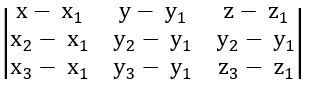The plane passing through the points (0, – 1, – 1), (4, 5, 1) and (3, 9, 4) is

x (30 – 20) – (y + 1) (20 – 6) + (z + 1) (40 – 18) = 0

x (10) – (y + 1) (14) + (z + 1) (22) = 0

10x – 14y – 14 + 22z + 22 = 0

10x – 14y + 22z + 8 = 0

2(5x – 7y + 11z + 4) = 0

∴ the equation of the plane is 5x – 7y + 11z + 4 = 0

##### Question 3

Find the equation to the plane parallel to the ZX-plane and passing through (0, 4, 4).

Sol:

Equation of ZX-plane is y = 0

The equation of the plane parallel to the ZX-plane is y = k

But it is passing through (0, 4, 4)

⟹ y = 4

###### Question 4

Find the equation to the plane passing through the point (α, β, γ) and parallel to the plane axe + by + cz + d = 0.

Sol:

The equation of the plane parallel to the plane ax + by + cz + d = 0 is ax + by + cz + k = 0

But it is passing through the point (α, β, γ)

a α + b β + c γ + k = 0

⟹ k = – a α – b β – c γ

The equation of the plane is ax + by + cz – a α – b β – c γ = 0

⟹ a(x – α) + b (y – β)+ c (z – γ) = 0

#### Question 5

Find the angle between the planes 2x – y + z = 6 and x + y + 2z = 7.

Sol: If θ is the angle between the planes a1 x + b1 y + c1 z + d1 = 0 and a2x + b2 y + c2 z + d2 = 0, then cos θ =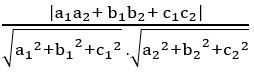Cos θ = cos 600

θ = 600 =

##### Question 6

Reduce the equation x + 2y – 2z – 9 = 0 to the normal form and hence find the dc’s of the normal to the plane.

Sol: Given plane is x + 2y – 2z – 9 = 0

x + 2y – 2z = 9

dividing into both sides by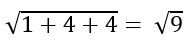dc’s of the normal to the plane are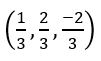##### Question 7

Suppose a plane makes intercepts 2, 3, 4 on X, Y, Z axes respectively. Find the equation of the plane in the intercept form.

Sol: Given a = 2, b = 3, c = 4

The equation of the line in the intercept form is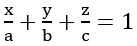#### Question 8

Express x – 3y + 2z = 9 in the intercept form

Sol: Given plane is x – 3y + 2z = 9

a = 9, b = – 3, c = 9/2

#### Question 9

Find the direction cosine of the normal to the plane x + 2y + 2z – 4 = 0.

Sol: Given plane is x + 2y + 2z – 4 = 0

We know that Dr’s of the normal to the plane ax + by + cz + d = 0 are (a, b, c)

⟹ dc’s of the normal to the plane =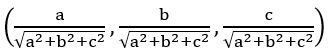⟹ dr’s of the normal to the plane x + 2y + 2z – 4 = 0 are (1, 2, 2)

⟹ dc’s of the normal to the plane are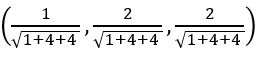##### Question 10

Find the midpoint of the line joining the points (1, 2, 3) and (–2, 4, 2)

Sol: Given points are A (1, 2, 3), B (–2, 4, 2)